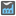• # 33 not factorizable?

## Question related to missionNumbers Factory

hi! in the description of exercise checkio(33)=0 , but i made a algorithm that factor 33 into 3x11...

```def is_prime(num):
count=0
for i in range(1,num+1):
if (num % i) == 0:
count += 1
if (count == 2):
return True
else:
return False

def get_lower_primes(num):
lowers=[]
for i in range(1,num):
if is_prime(i):
lowers.append(i)
return lowers

def factor(num):
#returns array of factor of a num
fac=[]
primes=get_lower_primes(num)
for i in range(len(primes)):
while (num % primes[i])==0:
fac.append(primes[i])
num = num // primes[i]
print fac

factor(33)
```

and returns [3, 11] I try to locate the error, but i couldn't, any help would be apreciatted ...